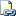# FLOOR (Transact-SQL)

Returns the largest integer less than or equal to the specified numeric expression.Transact-SQL Syntax Conventions

## Syntax

``````FLOOR ( numeric_expression )
``````

## Arguments

• numeric_expression
Is an expression of the exact numeric or approximate numeric data type category, except for the bit data type.

## Return Types

Returns the same type as numeric_expression.

## Examples

The following example shows positive numeric, negative numeric, and currency values with the `FLOOR` function.

``````SELECT FLOOR(123.45), FLOOR(-123.45), FLOOR(\$123.45)
``````

The result is the integer part of the calculated value in the same data type as numeric_expression.

``````---------      ---------     -----------
123            -124          123.0000
``````